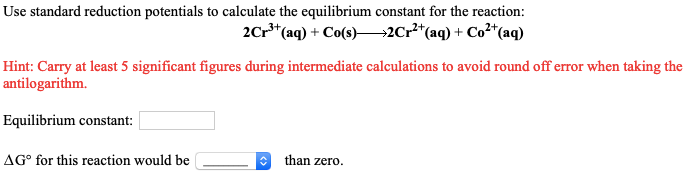# Use standard reduction potentials to calculate the equilibrium constant for the reaction: 2Cr^3+(aq) +Co(s) → 2Cr^2+(aq) + Co^2+(aq) Hint: Carry at least 5 significant figures during intermediate calculations to avoid round off error when taking the antilogarithm. ΔG° for this reaction would be ____ than zero.